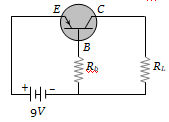The most commonly used material for making transistor is

(a) Copper                 (b) Silicon
(c) Ebonite                 (d) Silver

Concept Questions :-

Transistor
High Yielding Test Series + Question Bank - NEET 2020

Difficulty Level:

An NPN-transistor circuit is arranged as shown in figure. It is(a) A common base amplifier circuit
(b) A common emitter amplifier circuit
(c) A common collector amplifier circuit
(d) Neither of the above

Concept Questions :-

Transistor
High Yielding Test Series + Question Bank - NEET 2020

Difficulty Level:

The part of a transistor which is heavily doped to produce a large number of majority carriers is-

1. Base

2. Emitter

3. Collector

4. None of these

Concept Questions :-

Transistor
High Yielding Test Series + Question Bank - NEET 2020

Difficulty Level:

For a transistor, the current amplification factor is 0.8. The transistor is connected in common emitter configuration. The change in the collector current when the base current changes by 6 mA is
(a) 6 mA                       (b) 4.8 mA
(c) 24 mA                     (d) 8 mA

Concept Questions :-

Transistor
High Yielding Test Series + Question Bank - NEET 2020

Difficulty Level:

In a common base amplifier circuit, calculate the change in base current if that in the emitter current is 2 mA and $\mathrm{\alpha }$ = 0.98

(a) 0.04 mA                           (b) 1.96 mA
(c) 0.98 mA                           (d) 2 mA

Concept Questions :-

Transistor
High Yielding Test Series + Question Bank - NEET 2020

Difficulty Level:

In a transistor circuit shown here the base current is 35 $\mu$A. The value of the resistor Rb is(a) 123.5 k$\mathrm{\Omega }$
(b) 257 k$\mathrm{\Omega }$
(c) 380.05 k$\mathrm{\Omega }$
(d) None of these

Concept Questions :-

Transistor
High Yielding Test Series + Question Bank - NEET 2020

Difficulty Level:

For a transistor, in a common emitter arrangement, the alternating current gain $\mathrm{\beta }$ is given by

(a) $\mathrm{\beta }={\left(\frac{∆{\mathrm{I}}_{\mathrm{c}}}{∆{\mathrm{I}}_{\mathrm{B}}}\right)}_{{\mathrm{V}}_{\mathrm{C}}}$                       (b) $\mathrm{\beta }={\left(\frac{∆{\mathrm{I}}_{B}}{∆{\mathrm{I}}_{C}}\right)}_{{\mathrm{V}}_{\mathrm{C}}}$

(c) $\mathrm{\beta }={\left(\frac{∆{\mathrm{I}}_{\mathrm{c}}}{∆{\mathrm{I}}_{E}}\right)}_{{\mathrm{V}}_{\mathrm{C}}}$                       (d) $\mathrm{\beta }={\left(\frac{∆{\mathrm{I}}_{E}}{∆{\mathrm{I}}_{C}}\right)}_{{\mathrm{V}}_{\mathrm{C}}}$

Concept Questions :-

Transistor
High Yielding Test Series + Question Bank - NEET 2020

Difficulty Level:

The relation between $\mathrm{\alpha }$ and $\mathrm{\beta }$ parameters of current gains for a transistors is given by

(a) $\mathrm{\alpha }=\frac{\mathrm{\beta }}{1-\mathrm{\beta }}$                (b) $\mathrm{\alpha }=\frac{\mathrm{\beta }}{1+\mathrm{\beta }}$

(c) $\mathrm{\alpha }=\frac{1-\mathrm{\beta }}{\mathrm{\beta }}$                (d) $\mathrm{\alpha }=\frac{1+\mathrm{\beta }}{\mathrm{\beta }}$

Concept Questions :-

Transistor
High Yielding Test Series + Question Bank - NEET 2020

Difficulty Level:

In the CB mode of a transistor, when the collector voltage is changed by 0.5 volt. The collector current changes by 0.05 mA. The output resistance will be
(a) 10 k$\mathrm{\Omega }$                         (b) 20 k$\mathrm{\Omega }$
(c) 5 k$\mathrm{\Omega }$                           (d) 2.5 k$\mathrm{\Omega }$

Concept Questions :-

Transistor
High Yielding Test Series + Question Bank - NEET 2020

Difficulty Level:

Consider an NPN transistor amplifier in the common-emitter configuration. The current gain of the transistor is 100. If the collector current changes by 1 mA, what will be the change in emitter current?
(a) 1.1 mA                     (b) 1.01 mA
(c) 0.01 mA                    (d) 10 mA

Concept Questions :-

Transistor